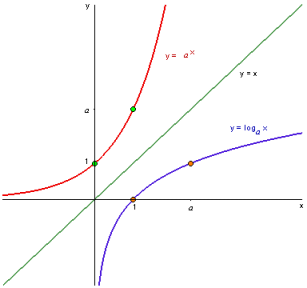# ML Wiki

## Exponential Functions

• $e^x$, or $\exp x$ is the exponential function
• Logarithm is inverse of exponent
•Base

• "Natural" base is $e = 2.718281828...$, where $e$ is the Euler's Number
• can be any other base: $a^x$

Algebraic Properties

• $e^x e^y = e^{x + y}$
• $(e^x)^y = e^{xy}$
• $\cfrac{d}{dx} e^x = e^x$
• $\int e^x\, dx = e^x + C$

## Taylor Expansion

Can expand $e^x = 1 + x + \cfrac{1}{2!}\, x^2 + \cfrac{1}{3!}\, x^3 + \cfrac{1}{4!}\, x^4 + \ ... \ = \sum\limits_{k=0}^{\infty} \cfrac{1}{k!} x^k$